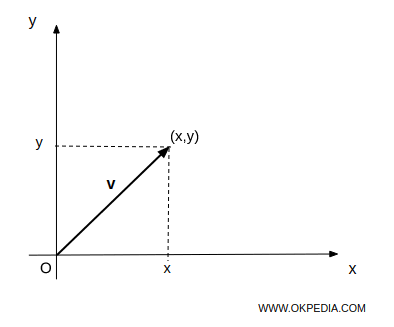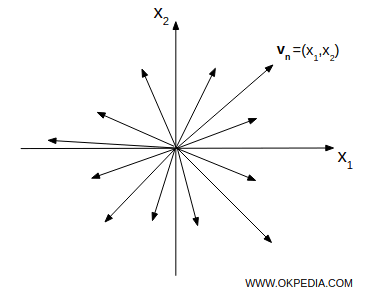## Vectors

Vectors are quantities characterized by a magnitude and a direction. In two or threedimensional space, a vector is represented by an arrow that has a certain length, an initial reference point (O) and an endpoint (x,y).## Vector space

A vector is a n-tuple of real number called components of vector. The set of all n-tuples of real numbers v = (x1,x2,...,xn) is called vector space n-dimensional or n-space and it's denoted by Rn.